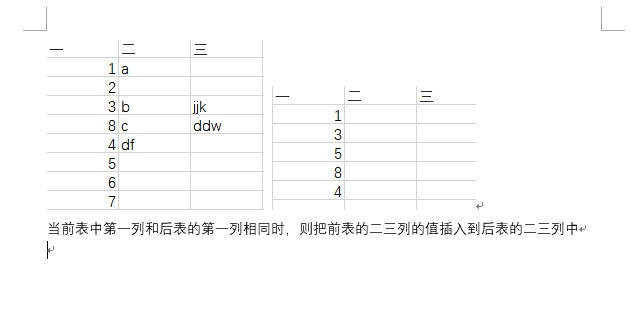sql插入数据的问题。求大神解答3个回答

update
tableB B
join
(select * from tabalA A join tableB B on A.colum1=B.colum1) t
on
B.colum1 = t.colum1
set B.colum2=t.colum2,B.colum3=t.colum3, where B.colum1= 1;
where条件不给定则会将B表全部update。u010618076 回复WWJ362429: 多谢了WWJ362429 回复u010618076: 把B表全部更新：update tableB B join (select * from tabalA A join tableB B on A.colum1=B.colum1) t on B.colum1 = t.colum1 set B.colum2=t.colum2,B.colum3=t.colum3u010618076 我要是就是想把B表全部update，那就不需要where子句了吧

update tableA a,tableB b
set b.col2=a.col2,b.col3=a.col3
where a.id=b.idu010618076 那这样是只更新tableA吗，会不会tableB也更新了

update
table2 b
inner join
table1 c
using(字段1)
set
b.字段2=c.字段2,
b.字段3=c.字段3